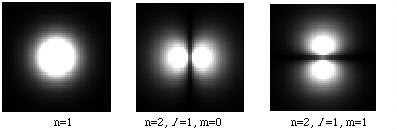# Quantum Numbers and the Periodic Table

We have seen that the energies of bound electrons are quantized, and have labelled the energy levels with an integer n. We mentioned that the orbits are not necessarily circular, nor are they planar. Instead, they are three dimensional, so that instead of circular, we have spherical, and instead of elliptical, we have ellipsoidal. In fact, the energy of an atomic electron depends not only on n, but on angular momentum, orientation, and spin as well. These effects are not predicted by the Bohr model, but derive from the more advanced (and complicated!) Schrodinger and Dirac Equations.

We therefore "label" an atomic electron by four "quantum numbers":

1. n = 1, 2, 3, ...., denoting energy;
2. l = 0, 1, ..., n-1, denoting angular momentum;
3. m = -l, ...., l, denoting orientation (the "magnetic quantum number"),
4. s = -1/2, 1/2, denoting spin (m s is also used in place of s).

The set of four numbers (n,l, m,s) identifies the "quantum state" of the electron. Chemists use letters in place of numbers for l (s, p, d, f, etc.), and so an n=3, l =1 electron would be denoted "3p".

The following images should give you a feel for what sorts of "orbits" these numbers correspond to. The images are cross sectional cuts of three dimensional orbits, so to get a complete picture you have to think of them as rotated around the vertical axis.Note that the orbits are not so much orbits as "shells". Current models describe the bound electrons not as particles but as fields; the shell is a sort of field density, which indicates the region which the electron occupies. The brightness in these images is proportional to the field density. So a bound electron is not a particle so much as a "fuzzy object". All of the Hydrogen orbitals for n = 1 to n = 4 are available in a quicktime movie (339K).

For multielectron atoms, it is almost always too complicated to solve the quantum equations exactly; this has only been done for Hydrogen and Helium (with recent progress on Lithium). Instead, we must resort to approximations and numerical computer analyses. The Bohr model holds for one electron atoms; for normal atoms, successively higher level electrons "see" successively lower values of Z due to "charge screening" of the nucleus by the inner electrons. The n = 1 electrons see the actual Z, while the outermost electron of a neutral atom sees Z = 1 (assuming n is large and l is zero).

When discussing multielectron atoms, we speak of "filling" the shells. By this we mean that we can assign electrons to shells by starting with the lowest quantum numbers and moving up in order until we reach the number of electrons in the atom (equal to Z for a neutral atom). The "Hartree Approximation" predicts the order in which electron orbits are filled, taking into account charge screening and the dependence of the energy on angular momentum which was ignored in the Bohr model. Since larger l values indicate that more of the electron field density is away from the nucleus, the screening is not spherical; the order of filling (and therefore of energy) is:

n, l
1,0
2,0 - 2,1
3,0 - 3,1
4,0 - 3,2 - 4,1
5,0 - 4,2 - 5,1
6,0 - 4,3 - 5,2 - 6,1
7,0 - 5,3 - 6,2

In fact, the energies depend not only on n and l , but to a much smaller degree on m and s as well, which means the above list is only approximately correct.

There is one more wrinkle, which remains one of the most extraordinary mysteries of physics. The "Pauli Principle" states that no two electrons may be in the same quantum state. This explains the chemical properties of the elements, as well as why "solid" objects are solid. We will see in the next chapter that most of the mass in the atom is concentrated in the nucleus, which is about five orders of magnitude smaller than the atom. The Coulomb repulsion of electrons in different objects is insufficient to keep them from passing through each other, but the electron fields can do so by the "magic" of not being allowed in the same place at the same time in the same state.

With this last piece of the model, we can build the Periodic Table of the Elements. The Periodic Table is based on the observation that an element's chemical properties depend on the number of electrons in its outer (valence) shell. Pauli's Principle means that we can only have two electrons for any given values of n, l and m; one has spin 1/2 and the other - 1/2. Similarly, for any given values of n and l , there can only be 2l +1 pairs of electrons, corresponding to the allowable values of m. For each of the pairs in the table above, you can count to see how many electrons are in the outer unfilled shell. Elements with the same number go in the same column. When the shell is full, the element goes in the (rightmost) "inert" column, since it does not easily react with a filled valence shell. The next element goes into the leftmost column (for those elements with only one electron in their outer shell).

Note that the energy levels used in constructing the Periodic Table are "ground" states (states of minimum energy). With sufficient energy (see the next section), any electron can "jump" to any level.